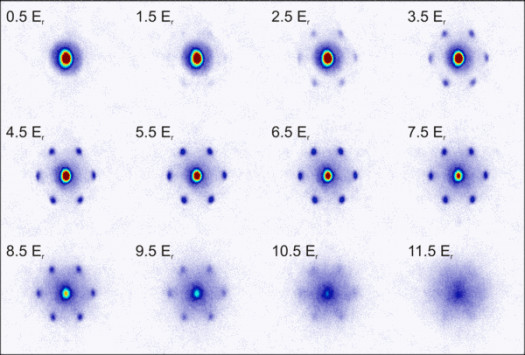# Do You Know Exponential Growth & Radicals?

10 Questions | Total Attempts: 102SettingsAn exponent refers to the number of times a number is multiplied by itself and In mathematics, a radical expression is defined as any expression containing a radical (√) symbol.

• 1.
In...., a radical expression is defined as any expression containing a radical symbol.
• A.

Mathematics

• B.

Music

• C.

Socioloigy

• D.

Biology

• 2.
Radical expressions is defined as any expression containing a radical symbol. What is the symbol?
• A.

• B.

• C.

• D.

>

• 3.
Exponential growth and radicals is a sub-topic under ...
• A.

Decimals

• B.

Indices

• C.

Algebra

• D.

Ratios

• 4.
Algebra is a topic in ....
• A.

Biology

• B.

Maths

• C.

Music

• D.

Literature

• 5.
Mathematics is usually taught at ...
• A.

Home

• B.

School

• C.

Musuem

• D.

Restaurant

• 6.
Who among the following people is not a mathematician?
• A.

Will Smith

• B.

Albert Einstein

• C.

Isaac Newton

• D.

Pythagoras

• 7.
3√(8) means to find the cube root of ....
• A.

1

• B.

2

• C.

3

• D.

8

• 8.
If there is no superscript number, the radical expression is calling for the ....
• A.

Square root

• B.

Cube root

• C.

Ratio

• D.

Decimals

• 9.
The term "radical" is derived from .... word.
• A.

Greek

• B.

Latin

• C.

Russian

• D.

American

• 10.
The first usage of the term was seen in England in the mid ....
• A.

1600s

• B.

1700s

• C.

1800s

• D.

1400s

Related TopicsBack to top